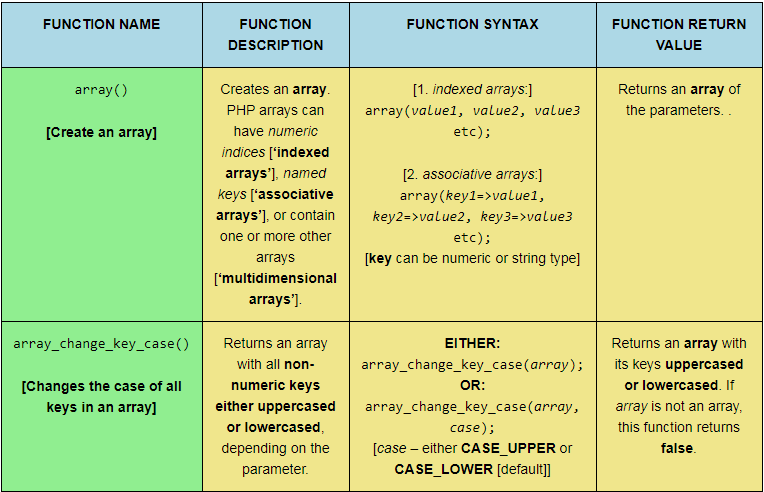# PHP Array Function Quiz: `array_diff_key()`; `array_diff_uassoc()`; `array_diff_ukey()`; `array_diff()`; `array_fill_keys()`; `array_fill()`; and `array_filter()`.This web page provides a reference table and a subsequent quiz to aid learning seven PHP array functions: `array_diff_key()`; `array_diff_uassoc()`; `array_diff_ukey()`; `array_diff()`; `array_fill_keys()`; `array_fill()`; and `array_filter()`. The quiz involves matching the function description, function syntax, and function return value to the correct function name. Different table formats are available in order to cater for users on a desktop computer, a laptop, or a mobile phone. Users can also choose to randomize the quiz elements as they see fit before clicking an ‘AUTO COMPLETE’ button and watching the computer correctly complete the quiz!

Tagged with: , , , ,
Posted in PHP Array Quizzes

# PHP Array Function Quiz: `array()`; `array_change_key_case()`; `array_chunk()`; `array_column()`; `array_combine()`; `array_count_values()`; and `array_diff_assoc()`.This web page provides a reference table and a subsequent quiz to aid learning seven PHP array functions: `array()`; `array_change_key_case()`; `array_chunk()`; `array_column()`; `array_combine()`; `array_count_values()`; and `array_diff_assoc()`. The quiz involves matching the function description, function syntax, and function return value to the correct function name. Different table formats are available in order to cater for users on a desktop computer, a laptop, or a mobile phone. Users can also choose to randomize the quiz elements as they see fit before clicking an ‘AUTO COMPLETE’ button and watching the computer correctly complete the quiz!

Tagged with: , , , ,
Posted in PHP Array Quizzes